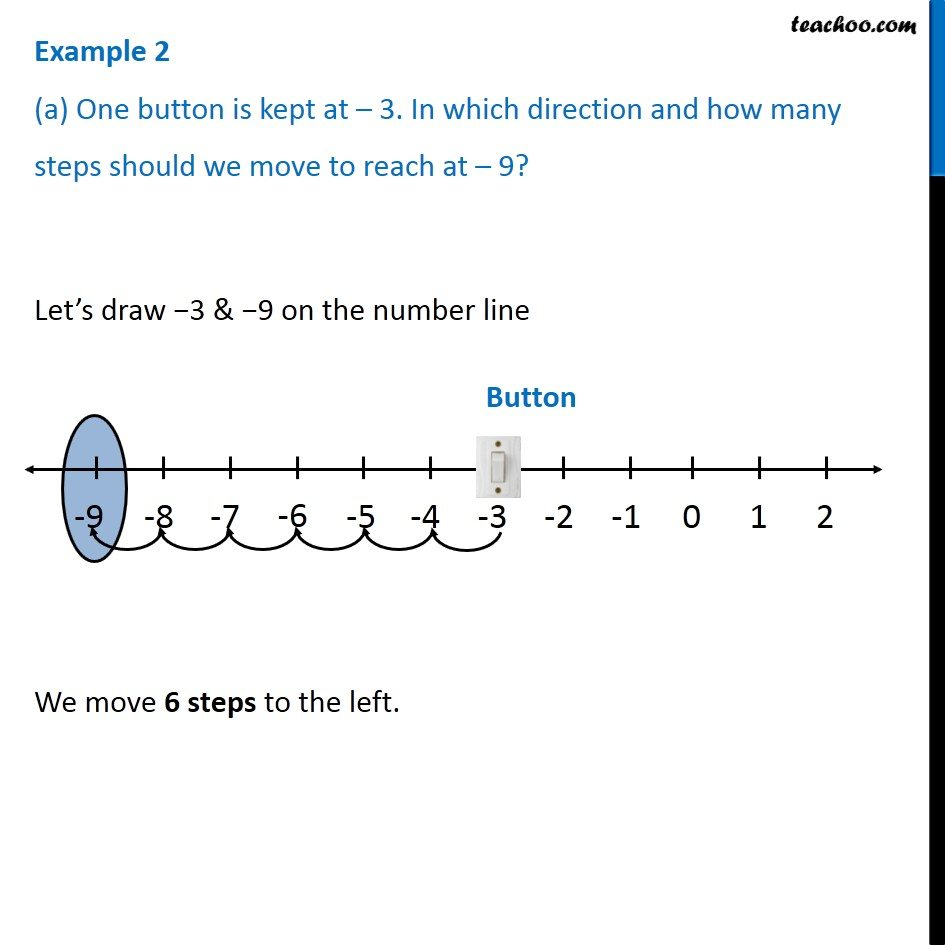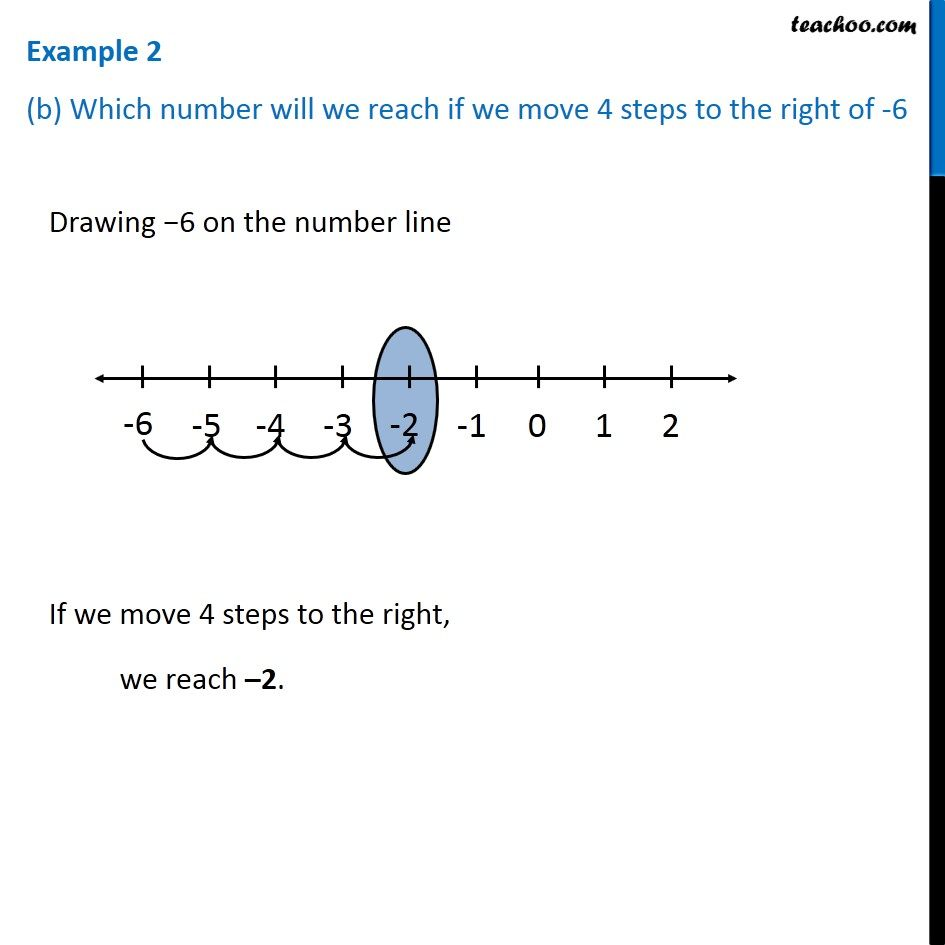1. Chapter 6 Class 6 Integers
2. Serial order wise
3. Examples

Transcript

Example 2 (a) One button is kept at 3. In which direction and how many steps should we move to reach at 9? Let s draw 3 & 9 on the number line We move 6 steps to the left. Example 2 (b) Which number will we reach if we move 4 steps to the right of -6Drawing 6 on the number line If we move 4 steps to the right, we reach 2.

Examples

Chapter 6 Class 6 Integers
Serial order wise